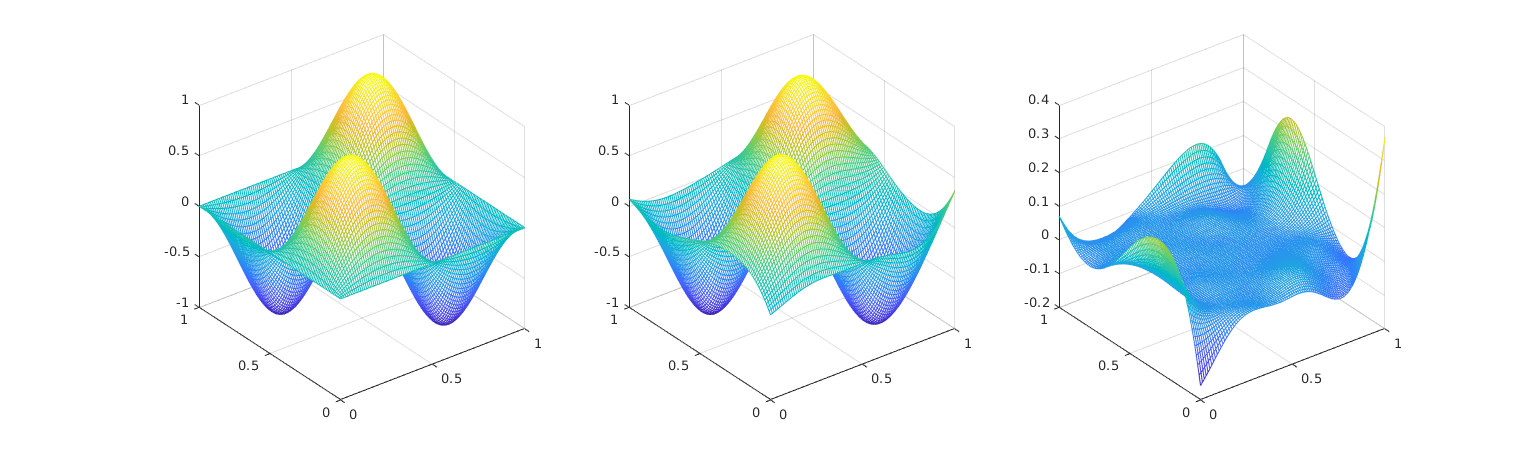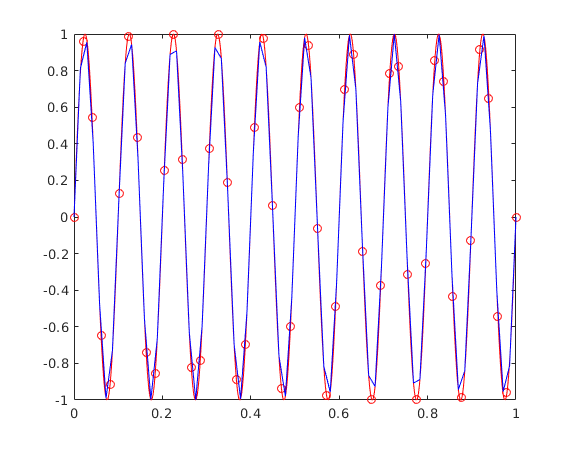# Radial Basis Functions Interpolation

Author: - 11 October 2017

This is a set of Matlab functions to interpolate scattered data with Radial Basis Functions (RBF).

## Getting Started

y = RBFinterp(xs, ys, x, RBFtype, R)


interpolates to find y, the values of the function y=f(x) at the points x.

Xs must be a matrix of size [N,Dx], with N the number of data points and Dx the dimension of the points in xs and x.

Ys must be a matrix of size [N,Dy], with N the number of known values at points in Xs, and Dy the dimension of the y values.

X must be a matrix of size [M,Dx], with M the number of query points.

RBFtype specifies the radial basis functions (RBF) to be used.

• The available global support RBFs are:
• ‘R1’ - linear spline
• ‘R3’ - cubic spline
• ‘TPS2’ - thin plate spline
• ‘Q’ - quadric
• ‘MQ’ - multiquadric
• ‘IMQ’ - inverse multiquadric
• ‘IQ’ - inverse quadric
• ‘GS’ - Gauss
RBF name Abbreviation
Linear spline R1
Cubic splie R3
Thin plate spline TPS2
Gauss GS
• The available compact support RBFs are (see Wendland H., Konstruktion und Untersuchung radialer Basisfunktionen mit kompaktem Träger. PhD thesis, Göttingen, Georg-August-Universität zu Göttingen, Diss, 1996):
• ‘CP_C0’
• ‘CP_C2’
• ‘CP_C4’
• ‘CP_C6’
• ‘CTPS_C0’
• ‘CTPS_C1’
• ‘CTPS_C2a’
• ‘CTPS_C2b’

Compact support functions have the form

RBF name

R is either the support radius for the compact support RBFs or a parameter to make the distance values dimensionless for the global support RBFs.

[fPar, M] = RBFparam(xs, ys, RBFtype, R)


returns the weights in the RBF summation and the polynomial coefficients in a column vector fPar by solving a linear system

[y] = RBFeval(xs, x, fPar, RBFtype, R)


returns the values of the interpolation weighted function at points x by performing the matrix-vector product

## Running the example

An example case can be run just by typing in the Matlab command line

test• Beckert, Armin and Wendland, Holger. Multivariate interpolation for fluid-structure-interaction problems using radial basis functions. Aerospace Science and Technology, 5 (2), p. 125-134, 2001.
• Wendland, Holger. Konstruktion und Untersuchung radialer Basisfunktionen mit kompaktem Träger}. PhD thesis, Göttingen, Georg-August-Universität zu Göttingen, Diss, 1996.
• De Boer, A and Van der Schoot, MS and Bijl, Hester. Mesh deformation based on radial basis function interpolation. Computers & structures, 85 (11-14), p. 784-795, 2007.
• Biancolini, Marco Evangelos. Fast Radial Basis Functions for Engineering Applications. Springer, 2018.